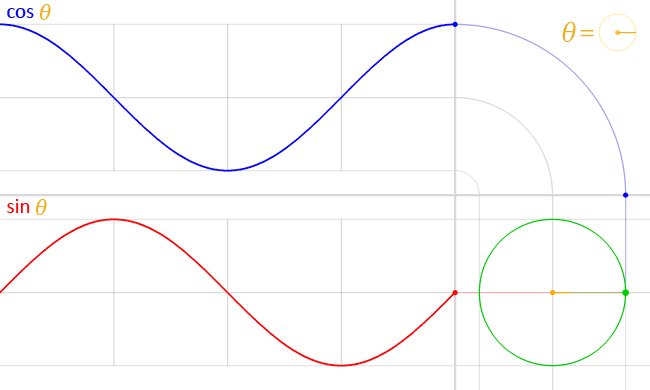The Art of Code is a MA/MFA class that I am teaching at Multidisciplinary New Media department in Paris College of Art. The course provides an introduction to coding for artists and designers who are willing to learn and use it as a form of creative expression.

This website documents
the course materials. If you have any questions, you can contact me on Twitter.

# Circular Motion

Circular motion is a movement in which an object travels along the circumference of a circle.

sketch is paused

``````
let radius;
let angle = 0;
let speed = 0.05;

function setup() {
createCanvas(400, 300);
radius = width / 6;
}

function draw() {
background(255);
translate(width/2, height/2);

// Empty Circle
noFill();
circle(0, 0, radius * 2);

// Rotating Circle
fill(0);
let x = cos(angle) * radius;
let y = sin(angle) * radius;
circle(x, y, 20);

// Increase angle every frame
angle += speed;
}
```
```

Working with circular motion requires a little bit of trigonometry knowledge so that we can convert between polar coordinate system and Cartesian coordinate system. If you haven't used trigonometry since school, you might need to brush up your skills. The following video from Khan Academy might help you get back on top of the basic concepts.

Below is a demo that shows how angles work in p5.js.

• Angle 0 is as shown below.
• Default `angleMode()` is `RADIANS`.
• A full circle is 360 `DEGREES`, which is equal to `TWO_PI` (2π) in `RADIANS`.
• Using `sin(angle) * radius`, we can calculate x coordinate of a point on the circumference of a circle.
• Using `cos(angle) * radius`, we can calculate y coordinate of the same point.

sketch is paused

As a result, sine and cosine are two numbers that oscillate between 1 and -1 according to angle change.There are a lot of possibilities for exciting, creative usages.

sketch is paused

Circular motion, simple harmonic motion, transverse waves are all related concepts. You can see in the demo below.

sketch is paused